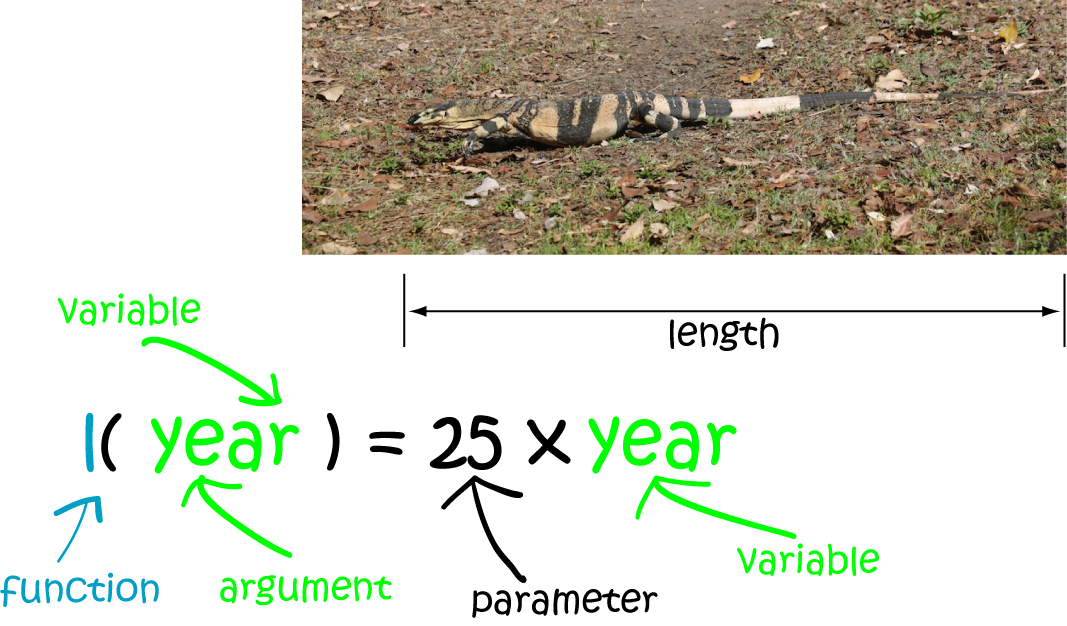# Definition of ParameterA parameter is a constant (or sometimes a variable) that forms part of a function, but can be changed so that the function works to solve another problem.

For example, in the length function for the goanna, the growth-rate parameter is $25$. This means that the goanna will grow $25\text{ cm}$ every year.

We could adapt this function to model the height of a tree that grows $20 \text{ cm}$ every year, for example, by changing the growth-rate parameter to $20$.

### Description

The aim of this dictionary is to provide definitions to common mathematical terms. Students learn a new math skill every week at school, sometimes just before they start a new skill, if they want to look at what a specific term means, this is where this dictionary will become handy and a go-to guide for a student.

### Audience

Year 1 to Year 12 students

### Learning Objectives

Learn common math terms starting with letter P

Author: Subject Coach
You must be logged in as Student to ask a Question.